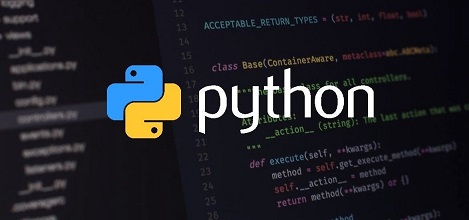# How to Remove Line from File Using Python

Sometimes you may need to remove lines from file. If you are using Linux, then you can easily do this using sed/awk command. But if you are not using Linux or want to do this from within your application or website, then it can be quite tedious to do this. In this article, we will learn how to remove line from file using Python.

## How to Remove Line from File Using Python

We will look at two use cases to remove line from file using python – one where the line index is unknown, and the other where it is known.

## 1. Position of line to be deleted is unknown

In this case, the position of line to be deleted is not known so we first open the file using open() command and read its contents into a python list using readlines() function.

Then we close and open the file again, to loop through the line one by one. We check each line to see if it is the line to be deleted and use write() function to write all lines except the one meant to be deleted. Let us say you have the following file sample.txt

```line1
line2
line3```

Here is the code to delete line containing string ‘line2’.

```#get list of lines
a_file = open("sample.txt", "r")

a_file.close()

new_file = open("sample.txt", "w")
for line in lines:

#delete line matching string
if line.strip("\n") != "line2":
new_file.write(line)

new_file.close()
```

Now if you print the file contents, you will see the following.

```line1
line3```

## 2. Position of Line to be deleted is known

In this case, we once again read the file content into a list using readlines(). But since we know the position of line to be deleted, we use del command and line position (as list index) to delete the list item from the list. Then we write back the updated list to the file. Here is the sample code to delete line #2 that is list index 1.

```#get list of lines
a_file = open("sample.txt", "r")

a_file.close()

#delete lines
del lines

#write file without line
new_file = open("sample.txt", "w+")

for line in lines:
new_file.write(line)

new_file.close()
```

In this article, we have learnt how to delete line from file using python. As you can see, it requires us to open files twice because, at the end of first opening, the file pointer reaches end of file. So you need to close the file and open it again to be able to write it one by one.